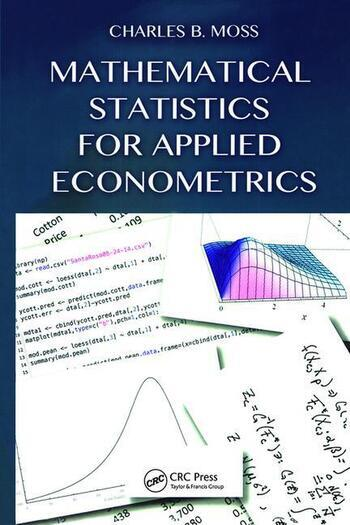# Mathematical Statistics for Applied Econometrics

## 1st Edition

Charles B Moss

Chapman and Hall/CRC
Published October 16, 2014
Textbook - 363 Pages - 54 B/W Illustrations
ISBN 9781466594098 - CAT# K20635

For Instructors Request Inspection Copy

was \$115.00

USD\$92.00

SAVE ~\$23.00

Currently out of stock
FREE Standard Shipping!

## Preview

### Summary

An Introductory Econometrics Text

Mathematical Statistics for Applied Econometrics covers the basics of statistical inference in support of a subsequent course on classical econometrics. The book shows students how mathematical statistics concepts form the basis of econometric formulations. It also helps them think about statistics as more than a toolbox of techniques.

Uses Computer Systems to Simplify Computation

The text explores the unifying themes involved in quantifying sample information to make inferences. After developing the necessary probability theory, it presents the concepts of estimation, such as convergence, point estimators, confidence intervals, and hypothesis tests. The text then shifts from a general development of mathematical statistics to focus on applications particularly popular in economics. It delves into matrix analysis, linear models, and nonlinear econometric techniques.

Students Understand the Reasons for the Results

Avoiding a cookbook approach to econometrics, this textbook develops students’ theoretical understanding of statistical tools and econometric applications. It provides them with the foundation for further econometric studies.

#### Instructors

We provide complimentary e-inspection copies of primary textbooks to instructors considering our books for course adoption.